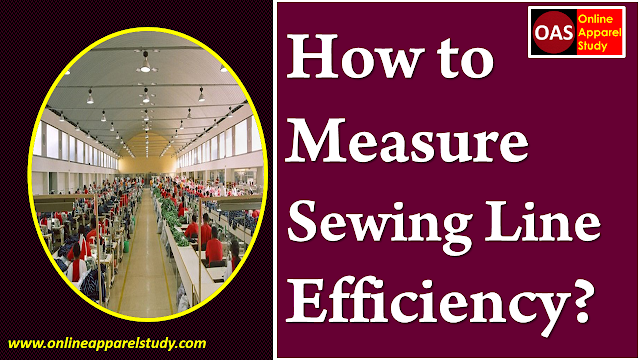## Sewing Line Efficiency

In the garments industry, efficiency is the ratio of produced minutes to spent minutes multiplied by 100. The formula to calculate Operator Efficiency, Sewing Line efficiency, Sewing Floor or Unit Efficiency and entire Factory Efficiency are the same. Just have to change some value to calculate the specific type of efficiency. In this article, we will learn how to calculate a Sewing Line Efficiency.Figure: Sewing Line Efficiency

## Sewing Line Efficiency Calculation

The common formula to calculate efficiency is-

Here,
Output                        = Number of garments pieces produced in the line
SMV                            = Standard Minute Value of the garment
Manpower                  = Number or the worker worked (Operator + Helper + ironman)
Working minute        = Total minute work to produce the garments.

Example:
A sewing line output is 1600 pcs in 9 hours. The operator was 32, the helper was 4 and the ironman was 2 and the garment SMV is 7.32. What is the efficiency of the sewing line?

Here,
Output                        = 1600 pcs
SMV                            = 7.32
Manpower                  = 32+4+2 = 38
Working minute        = 9 hours = 9 × 60 = 540 minutes

If we put all the value in the efficiency formula we will find the efficiency of that sewing line.
So, Sewing Line Efficiency = [(1600 × 7.32)/(38 × 540)] × 100 = 57.08%